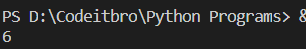# Python 3 Program to Generate A Random Number

4573In this tutorial, you will learn how to generate a random number in Python. If you are new to Python, then do check out this list of best Python books which you can refer to understand the basic concepts of Python programming language.

## Python 3 Program to Generate A Random Number

Generating random numbers in Python is quite simple. We will import the Random module to generate a random number between 0 to 100.  Refer to the code below.

### Source code

```# Program to generate a random number between 0 and 100
import random # importing the random module
print(random.randint(0,100))```

### Output